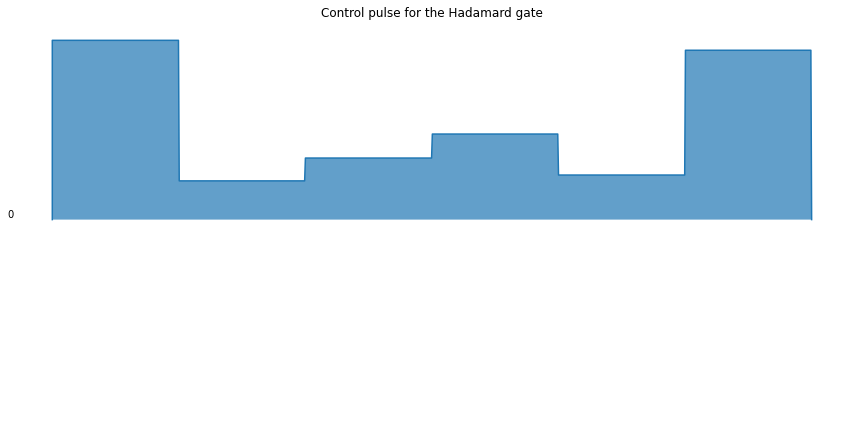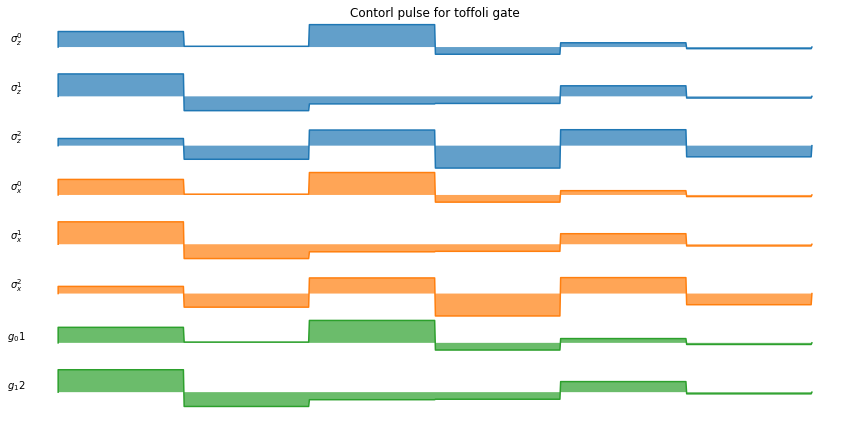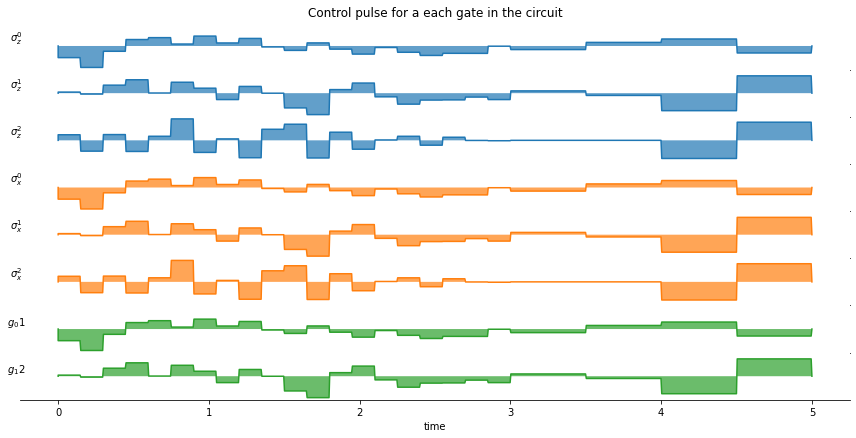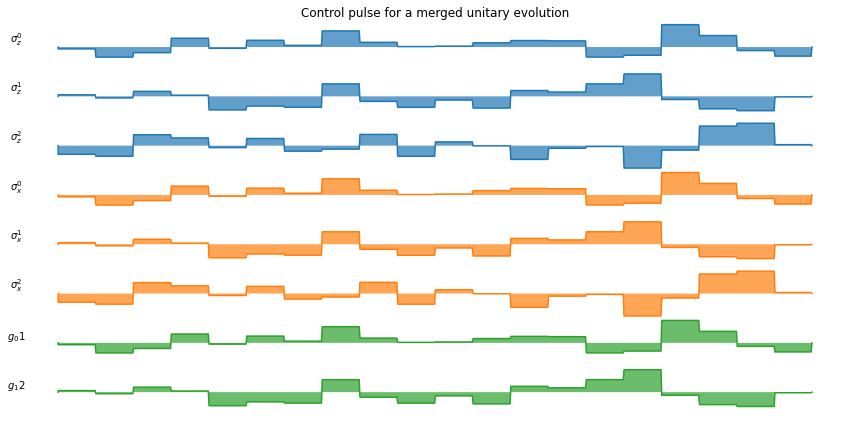# Examples for OptPulseProcessor¶

Author: Boxi Li ([email protected])

In :
from numpy import pi
from qutip import sigmaz, sigmax, identity, basis, fidelity, tensor
from qutip_qip.device import OptPulseProcessor
from qutip_qip.circuit import QubitCircuit
from qutip_qip.operations import expand_operator, toffoli


The qutip.OptPulseProcessor is a noisy quantum device simulator integrated with the optimal pulse algorithm from the qutip.control module. It is a subclass of qutip.Processor and is equipped with a method to find the optimal pulse sequence (hence the name OptPulseProcessor) for a qutip.QubitCircuit or a list of qutip.Qobj. For the user guide of qutip.Processor, please refer to the introductory notebook.

## Single-qubit gate¶

Like in the parent class Processor, we need to first define the available Hamiltonians in the system. The OptPulseProcessor has one more parameter, the drift Hamiltonian, which has no time-dependent coefficients and thus won't be optimized.

In :
num_qubits = 1
# Drift Hamiltonian
H_d = sigmaz()
# The (single) control Hamiltonian
H_c = sigmax()
processor = OptPulseProcessor(num_qubits, drift=H_d)


The method load_circuit calls qutip.control.optimize_pulse_unitary and returns the pulse coefficients.

In :
qc = QubitCircuit(num_qubits)

# This method calls optimize_pulse_unitary
num_tslots=6, evo_time=1, verbose=True)
processor.plot_pulses(title="Control pulse for the Hadamard gate", use_control_latex=False);

********** Gate 0 **********
Final fidelity error 6.248201955827426e-11
Terminated due to Goal achieved
Number of iterations 7Like the Processor, the simulation is calculated with a QuTiP solver. The method run_state calls mesolve and returns the result. One can also add noise to observe the change in the fidelity, e.g. the t1 decoherence time.

In :
rho0 = basis(2,1)
plus = (basis(2,0) + basis(2,1)).unit()
minus = (basis(2,0) - basis(2,1)).unit()
result = processor.run_state(init_state=rho0)
print("Fidelity:", fidelity(result.states[-1], minus))

processor.t1 = 40.0
result = processor.run_state(init_state=rho0)
print("Fidelity with qubit relaxation:", fidelity(result.states[-1], minus))

Fidelity: 1.0000000052177915
Fidelity with qubit relaxation: 0.9933419853438271


## Multi-qubit gate¶

In the following example, we use OptPulseProcessor to find the optimal control pulse of a multi-qubit circuit. For simplicity, the circuit contains only one Toffoli gate.

In :
toffoli()

Out:
Quantum object: dims = [[2, 2, 2], [2, 2, 2]], shape = (8, 8), type = oper, isherm = True\begin{equation*}\left(\begin{array}{*{11}c}1.0 & 0.0 & 0.0 & 0.0 & 0.0 & 0.0 & 0.0 & 0.0\\0.0 & 1.0 & 0.0 & 0.0 & 0.0 & 0.0 & 0.0 & 0.0\\0.0 & 0.0 & 1.0 & 0.0 & 0.0 & 0.0 & 0.0 & 0.0\\0.0 & 0.0 & 0.0 & 1.0 & 0.0 & 0.0 & 0.0 & 0.0\\0.0 & 0.0 & 0.0 & 0.0 & 1.0 & 0.0 & 0.0 & 0.0\\0.0 & 0.0 & 0.0 & 0.0 & 0.0 & 1.0 & 0.0 & 0.0\\0.0 & 0.0 & 0.0 & 0.0 & 0.0 & 0.0 & 0.0 & 1.0\\0.0 & 0.0 & 0.0 & 0.0 & 0.0 & 0.0 & 1.0 & 0.0\\\end{array}\right)\end{equation*}

We have single-qubit control $\sigma_x$ and $\sigma_z$, with the argument cyclic_permutation=True, it creates 3 operators each targeted on one qubit.

In :
N = 3
H_d = tensor([identity(2)] * 3)
test_processor = OptPulseProcessor(N, H_d)


The interaction is generated by $\sigma_x\sigma_x$ between the qubit 0 & 1 and qubit 1 & 2. expand_operator can be used to expand the operator to a larger dimension with given target qubits.

In :
sxsx = tensor([sigmax(),sigmax()])
sxsx01 = expand_operator(sxsx, 3, targets=[0,1])
sxsx12 = expand_operator(sxsx, 3, targets=[1,2])


Use the above defined control Hamiltonians, we now find the optimal pulse for the Toffoli gate with 6 time slots. Instead of a QubitCircuit, a list of operators can also be given as an input.

In :
def get_control_latex():
"""
Get the labels for each Hamiltonian.
It is used in the methodplot_pulses.
It is a 2-d nested list, in the plot,
a different color will be used for each sublist.
"""
return ([[r"$\sigma_z^%d$" % n for n in range(test_processor.num_qubits)],
[r"$\sigma_x^%d$" % n for n in range(test_processor.num_qubits)],
[r"$g_01$", r"$g_12$" ]])
test_processor.model.get_control_latex = get_control_latex

In :
test_processor.dims = [2,2,2]

In :
test_processor.load_circuit([toffoli()], num_tslots=6, evo_time=1, verbose=True);

test_processor.plot_pulses(title="Contorl pulse for toffoli gate");

********** Gate 0 **********
Final fidelity error 2.525584519297297e-08
Terminated due to function converged
Number of iterations 262## Merging a quantum circuit¶

If there are multiple gates in the circuit, we can choose if we want to first merge them and then find the pulse for the merged unitary.

In :
qc = QubitCircuit(3)

In :
setting_args = {"CNOT": {"num_tslots": 20, "evo_time": 3},
"RX": {"num_tslots": 2, "evo_time": 1},
"RY": {"num_tslots": 2, "evo_time": 1}}

fig, axes = test_processor.plot_pulses(title="Control pulse for a each gate in the circuit", show_axis=True);
axes[-1].set_xlabel("time");

********** Gate 0 **********
Final fidelity error 9.288031862508817e-07
Terminated due to function converged
Number of iterations 351
********** Gate 1 **********
Final fidelity error 3.309241769500204e-12
Terminated due to Goal achieved
Number of iterations 8
********** Gate 2 **********
Final fidelity error 4.594247204892099e-11
Terminated due to Goal achieved
Number of iterations 28In the above figure, the pulses from $t=0$ to $t=3$ are for the CNOT gate while the rest for are the two single qubits gates. The difference in the frequency of change is merely a result of our choice of evo_time. Here we can see that the three gates are carried out in sequence.

In :
qc = QubitCircuit(3)
test_processor.plot_pulses(title="Control pulse for a merged unitary evolution");

********** Gate 0 **********
Final fidelity error 1.3890985953723956e-06
Terminated due to function converged
Number of iterations 354In this figure there are no different stages, the three gates are first merged and then the algorithm finds the optimal pulse for the resulting unitary evolution.

In :
import qutip_qip
print("qutip-qip version:", qutip_qip.version.version)
from qutip.ipynbtools import version_table
version_table()

qutip-qip version: 0.2.0

Out:
SoftwareVersion
QuTiP4.6.3
Numpy1.22.2
SciPy1.8.0
matplotlib3.5.1
Cython0.29.27
Number of CPUs12
BLAS InfoOPENBLAS
IPython8.0.1
Python3.9.0 | packaged by conda-forge | (default, Nov 26 2020, 07:53:15) [MSC v.1916 64 bit (AMD64)]
OSnt [win32]
Thu Feb 10 23:43:00 2022 W. Europe Standard Time
In [ ]: